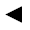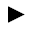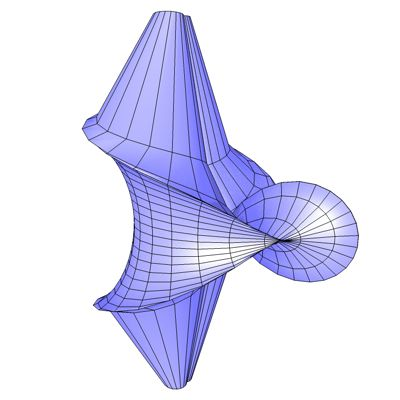[back]

## 3.51. Kuen's Surface

[next]The Kuen's Surface [ 2,7,11 ] is represented by the following equations. To keep the formulas a little clearer, we use the constant h.

 h = 1 + u 2 sin(v) sin(v) 3-168 x = (2 (cos(u) + u sin(u)) sin(v))/h 3-169 y = (2 (-u cos(u) + sin(u)) sin(v))/h 3-170 z = log(tan(v/2)) + 2 cos(v)/h 3-171

To represent the area, the two parameters u and v can assume the following values ​​(domain of definition), for example.

 u is an element from the set of numbers [-4.3, 4.3] v is an element of the number set [0.03, 3.11]

The Kuen's Surface is an open figure, so the definition range can be changed with the plugin.Fig. 55